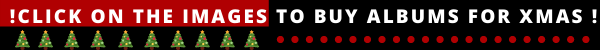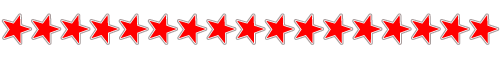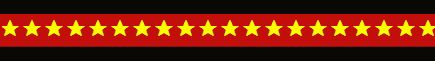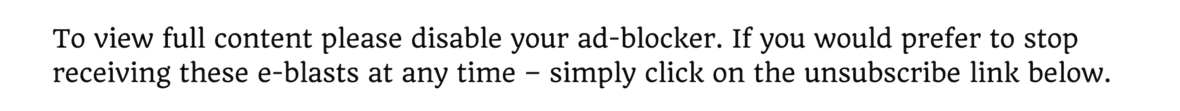Like   Tweet   Pin   +1   intable.module-2{width:99.62%;padding:0}table div table+table+table div table{width:99.62%;float:none;margin-left:auto;margin-right:auto;padding:0}table div table+table+table div table a{border:0 none;text-decoration:none}table div table+table+table div table img{width:100%!important;border:0 none;text-decoration:none}table div table+table+table div table td{width:100%;padding:0}/* styles */
 table.module-3{width:99.62%;padding:0}table div table+table+table+table div table{width:99.62%;float:none;margin-left:auto;margin-right:auto;padding:0}table div table+table+table+table div table a{border:0 none;text-decoration:none}table div table+table+table+table div table img{width:100%!important;border:0 none;text-decoration:none}table div table+table+table+table div table td{width:100%;padding:0}/* styles */
 table.module-4{width:99.62%;padding:0}table div table+table+table+table+table div table{width:99.62%;float:none;margin-left:auto;margin-right:auto;padding:0}table div table+table+table+table+table div table a{border:0 none;text-decoration:none}table div table+table+table+table+table div table img{width:100%!important;border:0 none;text-decoration:none}table div table+table+table+table+table div table td{width:100%;padding:0}/* styles */
 table.module-5{width:99.62%;padding:0}table div table+table+table+table+table+table div table{width:99.62%;float:none;margin-left:auto;margin-right:auto;padding:0}table div table+table+table+table+table+table div table a{border:0 none;text-decoration:none}table div table+table+table+table+table+table div table img{width:100%!important;border:0 none;text-decoration:none}table div table+table+table+table+table+table div table td{width:100%;padding:0}/* styles */
 table.module-6{width:99.62%;padding:0}table div table+table+table+table+table+table+table div table{width:99.62%;float:none;margin-left:auto;margin-right:auto;padding:0}table div table+table+table+table+table+table+table div table a{border:0 none;text-decoration:none}table div table+table+table+table+table+table+table div table img{width:100%!important;border:0 none;text-decoration:none}table div table+table+table+table+table+table+table div table td{width:100%;padding:0}/* styles */
 table.module-7{width:99.62%;padding:0}table div table+table+table+table+table+table+table+table div table{width:99.62%;float:none;margin-left:auto;margin-right:auto;padding:0}table div table+table+table+table+table+table+table+table div table a{border:0 none;text-decoration:none}table div table+table+table+table+table+table+table+table div table img{width:100%!important;border:0 none;text-decoration:none}table div table+table+table+table+table+table+table+table div table td{width:100%;padding:0}/* styles */
 table.module-8{width:99.62%;padding:0}table div table+table+table+table+table+table+table+table+table div table{width:99.62%;float:none;margin-left:auto;margin-right:auto;padding:0}table div table+table+table+table+table+table+table+table+table div table a{border:0 none;text-decoration:none}table div table+table+table+table+table+table+table+table+table div table img{width:100%!important;border:0 none;text-decoration:none}table div table+table+table+table+table+table+table+table+table div table td{width:100%;padding:0}/* styles */
 table.module-9{width:99.62%;padding:0}table div table+table+table+table+table+table+table+table+table+table div table{width:99.62%;float:none;margin-left:auto;margin-right:auto;padding:0}table div table+table+table+table+table+table+table+table+table+table div table a{border:0 none;text-decoration:none}table div table+table+table+table+table+table+table+table+table+table div table img{width:100%!important;border:0 none;text-decoration:none}table div table+table+table+table+table+table+table+table+table+table div table td{width:100%;padding:0}/* styles */
 table.module-10{width:99.62%;padding:0}table div table+table+table+table+table+table+table+table+table+table+table div table{width:99.62%;float:none;margin-left:auto;margin-right:auto;padding:0}table div table+table+table+table+table+table+table+table+table+table+table div table a{border:0 none;text-decoration:none}table div table+table+table+table+table+table+table+table+table+table+table div table img{width:100%!important;border:0 none;text-decoration:none}table div table+table+table+table+table+table+table+table+table+table+table div table td{width:100%;padding:0}/* styles */
 table.module-11{width:99.62%;padding:0}table div table+table+table+table+table+table+table+table+table+table+table+table div table{width:99.62%;float:none;margin-left:auto;margin-right:auto;padding:0}table div table+table+table+table+table+table+table+table+table+table+table+table div table a{border:0 none;text-decoration:none}table div table+table+table+table+table+table+table+table+table+table+table+table div table img{width:100%!important;border:0 none;text-decoration:none}table div table+table+table+table+table+table+table+table+table+table+table+table div table td{width:100%;padding:0}/* styles */
 table.module-12{width:99.62%;padding:0}table div table+table+table+table+table+table+table+table+table+table+table+table+table div table{width:99.62%;float:none;margin-left:auto;margin-right:auto;padding:0}table div table+table+table+table+table+table+table+table+table+table+table+table+table div table a{border:0 none;text-decoration:none}table div table+table+table+table+table+table+table+table+table+table+table+table+table div table img{width:100%!important;border:0 none;text-decoration:none}table div table+table+table+table+table+table+table+table+table+table+table+table+table div table td{width:100%;padding:0}/* styles */
 table.module-13{width:99.62%;padding:0}table div table+table+table+table+table+table+table+table+table+table+table+table+table+table div table{width:99.62%;float:none;margin-left:auto;margin-right:auto;padding:0}table div table+table+table+table+table+table+table+table+table+table+table+table+table+table div table a{border:0 none;text-decoration:none}table div table+table+table+table+table+table+table+table+table+table+table+table+table+table div table img{width:100%!important;border:0 none;text-decoration:none}table div table+table+table+table+table+table+table+table+table+table+table+table+table+table div table td{width:100%;padding:0}/* styles */
 table div table+table+table+table+table+table+table+table+table+table+table+table+table+table+table div table td,table.module-14{width:100%;padding:0}table div table+table+table+table+table+table+table+table+table+table+table+table+table+table+table div table{width:100%;float:none;margin-left:auto;margin-right:auto;padding:0}table div table+table+table+table+table+table+table+table+table+table+table+table+table+table+table div table a{border:0 none;text-decoration:none}table div table+table+table+table+table+table+table+table+table+table+table+table+table+table+table div table img{width:100%!important;border:0 none;text-decoration:none}/* styles */Reach out to promoters and beyond with your message, photos and videos via Raestar's Promotions | Danielle White +44 (0)7841 675 263| daniraestar@aol.com www.daniraestar.com #BLACKLIVESMATTER
AUTUMN JAZZ SHOWCASE
LATEST FEATURE E-BLAST
JAZZ VIDEO SHOWCASE VOLUME 1